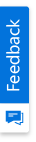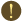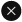We use cookies to give you the best experience on our website. If you continue to browse, then you agree to our privacy policy and cookie policy.Unfortunately, activation email could not send to your email. Please try again.# Blazor 100% Stacked Line Chart – Beautiful, Easy-to-Use Chart

## Overview

Blazor 100% Stacked Line Chart displays multiple series of data, ensuring that the cumulative proportion of each stacked element always totals 100%. Hence, the y-axis will always be rendered with the range 0–100.## Marker

Marks data points with built-in shapes such as circles, rectangles, ellipses, vertical lines, horizontal lines, diamonds, triangles, and pentagons. In addition to these shapes, use images to make the point more attractive.## Data label

Data labels display information about data points. Add a template to display data labels with HTML elements such as images, DIV, and spans for more informative data labels. You can rotate a data label by its given angle.## Multiple axes

Use multiple axes to plot different data sets that widely vary from one other.## Customization

Customize the look and feel of the Blazor 100% Stacked Line Chart using built-in APIs.

Customization documentation## Blazor 100% Stacked Line Chart Code Example

Easily get started with Blazor 100% Stacked Line Chart using a few simple lines of C# code, as demonstrated below. Also explore our Blazor 100% Stacked Line Chart Example that shows you how to render and configure the chart.

```@using Syncfusion.Blazor.Charts

<SfChart>
<ChartSeries XName="X" Width="2" DashArray="5,1" DataSource="@ExpenseReports" YName="Y" Type="ChartSeriesType.StackingLine100">
<ChartMarker Visible="true"/>
</ChartSeries>
<ChartSeries XName="X" Width="2" DashArray="5,1" DataSource="@ExpenseReports" YName="Y1" Type="ChartSeriesType.StackingLine100">
<ChartMarker Visible="true"/>
</ChartSeries>
<ChartSeries XName="X" Width="2" DashArray="5,1" DataSource="@ExpenseReports" YName="Y2" Type="ChartSeriesType.StackingLine100">
<ChartMarker Visible="true"/>
</ChartSeries>
<ChartSeries XName="X" Width="2" DashArray="5,1" DataSource="@ExpenseReports" YName="Y3" Type="ChartSeriesType.StackingLine100">
<ChartMarker Visible="true"/>
</ChartSeries>
</SfChart>

@code
{
public class ChartData
{
public string X { get; set; }
public double Y { get; set; }
public double Y1 { get; set; }
public double Y2 { get; set; }
public double Y3 { get; set; }
}
public List<ChartData> ExpenseReports = new List<ChartData>
{
new ChartData { X = "Food" , Y = 90, Y1 = 40 , Y2= 70, Y3= 120},
new ChartData { X = "Transport", Y = 80, Y1 = 90, Y2= 110, Y3= 70 },
new ChartData { X = "Medical",Y = 50, Y1 = 80, Y2= 120, Y3= 50 },
new ChartData { X = "Clothes",Y = 70, Y1 = 30, Y2= 60, Y3= 180 },
new ChartData { X = "Personal Care", Y = 30, Y1 = 80, Y2= 80, Y3= 30 },
new ChartData { X = "Books", Y = 10, Y1 = 40, Y2= 30, Y3= 270},
new ChartData { X = "Fitness",Y = 100, Y1 = 30, Y2= 70, Y3= 40 },
new ChartData { X = "Electricity", Y = 55, Y1 = 95, Y2= 55, Y3= 75},
new ChartData { X = "Tax", Y = 20, Y1 = 50, Y2= 40, Y3= 65 },
new ChartData { X = "Pet Care", Y = 40, Y1 = 20, Y2= 80, Y3= 95 },
new ChartData { X = "Education", Y = 45, Y1 = 15, Y2= 45, Y3= 195 },
new ChartData { X = "Entertainment", Y = 75, Y1 = 45, Y2= 65, Y3= 115 }
};
}```

## Not sure how to create your first Blazor 100% Stacked Line Chart? Our documentation can help.

### Blazor Components – 80+ UI and DataViz Components

ALL COMPONENTS
FILE VIEWERS & EDITORS
DOCUMENT PROCESSING LIBRARIES
REPORTSYou are using an outdated version of Internet Explorer that may not display all features of this and other websites. Upgrade to Internet Explorer 8 or newer for a better experience.